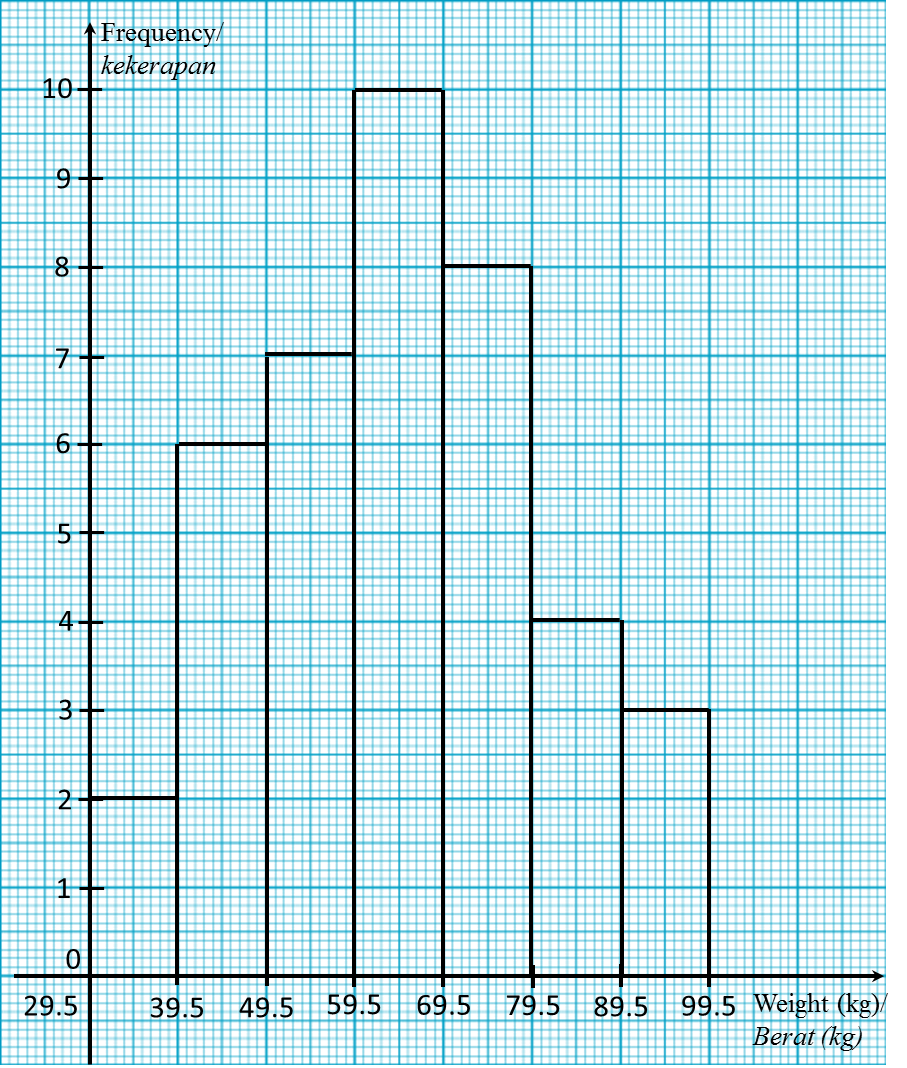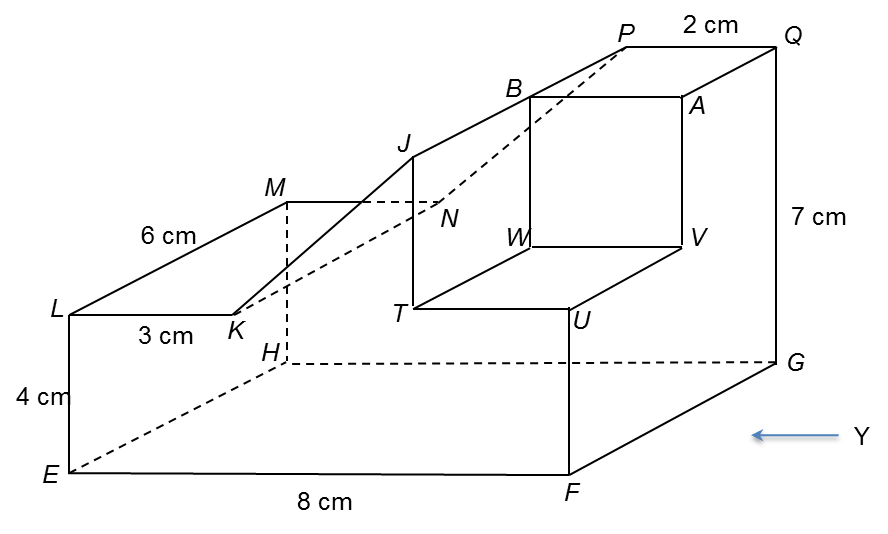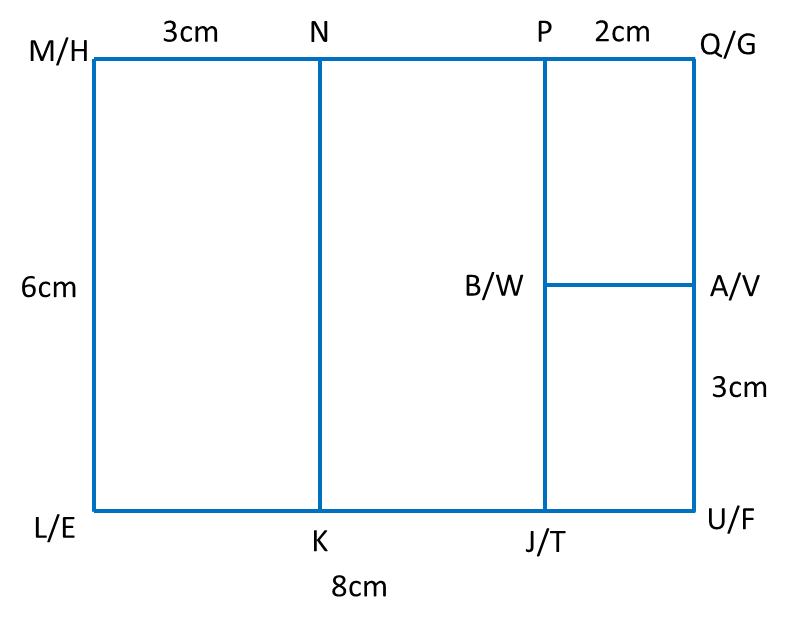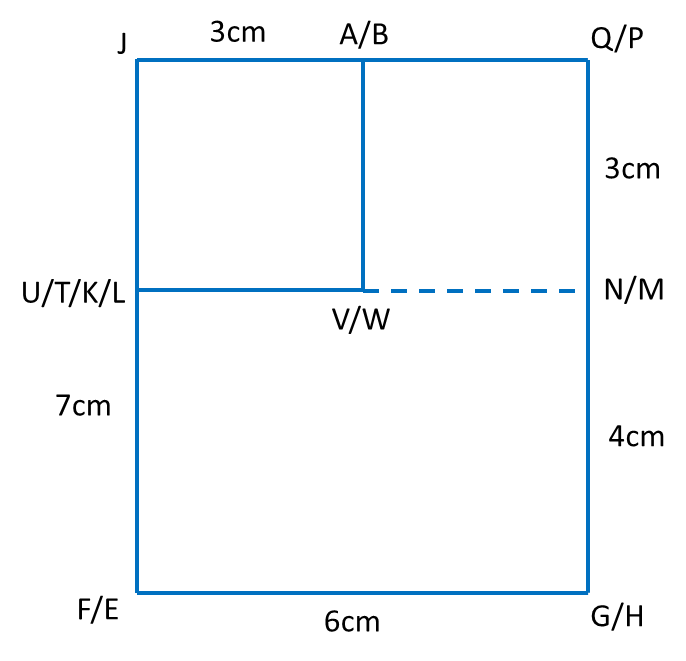# SPM Mathematics (Model Test Paper), Question 14 & 15

SPM Mathematics (Model Test Paper)

Section B
[48 marks]
Answer any four questions from this section.

14.  The data in Diagram 9 shows the weight of meat, in kg, sold each day over 40 days.

 36 63 43 47 67 71 68 79 92 49 41 52 66 78 84 55 77 86 58 94 60 74 73 62 56 68 46 35 72 68 80 40 57 59 71 81 92 60 63 53
Diagram 9

(a) Based on the data in Diagram 9, complete Table 14 in the answer space. [ 4 marks]
(b) Based on Table 2, calculate the estimated mean of the weight of meat sold over 40 days. [3 marks]
(c) For this part of question, use the graph paper provided on page. By using the   scale of 2 cm to 10 kg on the horizontal axis and 2 cm to 1 day on the vertical axis, draw a histogram for the data. [4 marks]
(d) Based on the histogram  in 14(c), state one information about the weight of meat sold each day. [1 mark]

Answer:
(a)
 Weight (kg) Mid point Frequency 30 – 39
Table 2

Answer and solution:
(a)

 Weight (kg) Mid point  (x) Frequency (f) 30 – 39 34.5 2 40 – 49 44.5 6 50 – 59 54.5 7 60 – 69 64.5 10 70 – 79 74.5 8 80 – 89 84.5 4 90 – 99 94.5 3

(b)

(c)(d)
Modal class is 60 – 69.

15.  You are not allowed to use graph paper to answer this question.
(a)  Diagram  10.1  shows a solid right prism with rectangular base EFGH  on a horizontal table.  The surface EFRJKL  is the uniform cross-section of the prism. Rectangle KLMN  and JPQR are horizontal planes.  Rectangle  JKNP  is an inclined plane. RF and LE  are vertical edges.Diagram 10.1

Draw full scale, the elevation of the solid on a vertical plane parallel to EF as viewed from  X    [3 marks]
(b)  A solid cuboid is removed from the solid in Diagram 10.1. The remaining solid is as shown in Diagram 10.2. Rectangle TUVW is a horizontal plane. JT and BW are vertical edges. UF = 4 cm and UV= 3 cm.Diagram 10.2
Draw to full scale,
(i) the plan of the remaining solid. [4 marks]
(ii) the elevation of the remaining solid on a vertical plane parallel to FG  as viewed from Y. [5 marks]

Answer and solution:
(a)(b)(i)(b)(ii)